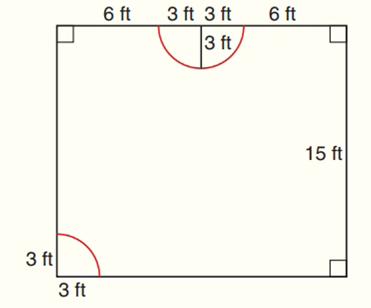Chapter 8.CR, Problem 39CR### Elementary Geometry for College St...

6th Edition
Daniel C. Alexander + 1 other
ISBN: 9781285195698

#### Solutions

Chapter
Section### Elementary Geometry for College St...

6th Edition
Daniel C. Alexander + 1 other
ISBN: 9781285195698
Textbook Problem
1 views

# Jeff and Helen want to carpet their family room, except for the entrance way and the semicircle in front of the fireplace, both of which they want to tile.a) How many square yards of carpeting are needed?b) How many square feet are to be tiled?To determine

(a)

To find:

The number of square yards of carpeting are needed approximately.

Explanation

Theorem:

In a circle of radius length r, the area A of a sector whose arc has degree measure m is given by

A=m360πr2.

Postulate:

The area A of a rectangle whose base has length b and whose altitude has length h is given by A=bh.

Formula:

The area of the semi-circle A=12πr2.

Calculation:

Given that Jeff and Helen want to carpet their family room, except for the entrance way and the semicircle in front of the fireplace, both of which they want to tile.

We need to find out the number of square yards of carpeting are needed.

The area of carpeting needed = the area of rectangle – the area of semi-circle – the area of entry way which is in the sector shape.

To find the area of rectangle:

A=bh

Base (b) = 6ft + 3ft + 3ft + 6ft = 18ft

Height (h) = 15ft

A=15×18=270

So, the area of rectangle =270 ft2.

To find the area of semi-circle:

A=12πr2

A=12π(3)2=9×3

To determine

(b)

To find:

The number of square feet are to be tiled.

### Still sussing out bartleby?

Check out a sample textbook solution.

See a sample solution

#### The Solution to Your Study Problems

Bartleby provides explanations to thousands of textbook problems written by our experts, many with advanced degrees!

Get Started

#### Calculate y'. 21. y = 3x ln x

Single Variable Calculus: Early Transcendentals

#### Show that the equation has exactly one real root. x3 + ex = 0

Single Variable Calculus: Early Transcendentals, Volume I

#### The vector represented by where A(4, 8) and B(6, 6)is:

Study Guide for Stewart's Multivariable Calculus, 8th